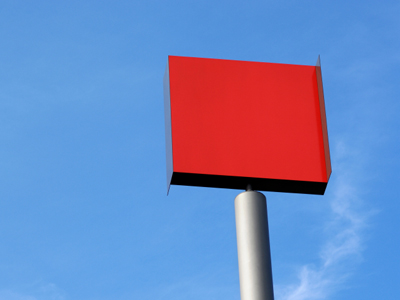Find out why 81 is a square number in this quiz.

# Properties of Numbers (Medium)

'Odd' and 'even' are properties of numbers. Do this 11-plus Maths quiz and see how much you know about numbers.

You might think it's not important to know that 2, 4 and 6 are even numbers. This is true when doing simple calculations such as 6 + 3. Whether they are odd or even has little bearing on the sum. However, if you want to go into a career where a thorough understanding of maths is vital ~ and there are plenty of them ~ you'll need to know a lot more about numbers than how to do basic calculations.

The more you know about numbers, the more you will understand them and the better you will be when working with them.

Are you ready to play the quiz? OK then ~ 10, 9, 8, 7, 6, 5, 4, 3, 2, 1 ~ off you go!

1.
Which of the following statements is wrong?
For every positive number there is a negative number
There are more positive numbers than negative numbers
The smallest non negative even number is 2
The smallest non negative odd number is 1
Not so! For every positive number there is a negative number, e.g. 2 and -2, 1,000 and -1,000, 3.4 and -3.4: this goes on and on without end for every number you care to think of
2.
What do you always get when you add a positive whole number and a negative whole number?
Either a positive or negative number
A negative number
A positive number
Either a positive number or negative number or zero
For example: 6 + -5 = 1 (a positive number); 6 + -7 = -1 (a negative number); 6 + -6 = 0 (zero). DON'T forget zero - it is a number
3.
If a number is divisible by 10, what must the final digit of the number be?
1
5
0
4
You can cancel the zero in the number with the zero in ten and write the answer straight away, e.g. 120 ÷ 10 = 12
4.
How can an odd number always be made into an even number?
Subtract 2 from it
Divide it by 2
Multiply it by 2
Doubling an odd number will always produce an even number
5.
Which words do not describe the numbers 3, 5, 7 and 9?
Even numbers
Odd numbers
Whole numbers
Each number is two more than the previous number
They are all whole odd numbers, and each number is two more than then previous number
6.
What is meant by the following statement: 48 is a multiple of 4?
Four has to be multiplied 48 times with itself
Four is the square of 48
Four goes into 48 exactly
Four does not go into 48 exactly
If a number is a multiple of another number, then it is divisible by the other number exactly a certain number of times, e.g. 4 goes into 48 exactly 12 times because 12 × 4 = 48. Note: 16.8 is a multiple of 2.8 because 2.8 × 6 = 16.8: so the idea of 'multiples' also applies to decimal numbers
7.
Which of the following is not a square number?
64
48
121
81
A square number is formed by multiplying another number with itself : 64 = 8 × 8; 121 = 11 × 11; 81 = 9 × 9. Note: 1 × 1 = 1
8.
If a number is divisible by 5, what must the final digit of the number be?
0 or 5
5 only
0 only
1 only
For example, 130 (ends in zero) and 345 (ends in five) are both divisible by 5
9.
Which words do not describe the numbers 2, 4, 6 and 8?
Odd numbers
Even numbers
Whole numbers
Multiples of two
They are all whole even numbers and multiples of 2
10.
Which of the following statements is wrong?
If a number is a multiple of 8, it is divisible by both 2 and 4
If a number is divisible by 2, it is an even number
If a number is divisible by 2, it is divisible by 4
If a number is divisible by 4, it is divisible by 2
Not so, e.g. 2 × 9 = 18 BUT 18 is NOT divisible by 4. If you want to show that a statement is not always true, find one example that shows it is wrong - like we did here
Author:  Frank Evans